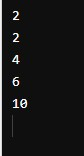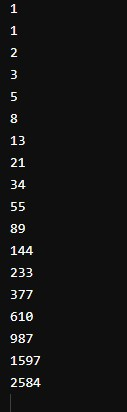# Fibonacci Sequence in Ruby

We will introduce the Fibonacci sequence in Ruby with examples.

## Fibonacci Sequence in Ruby

We may need to get a range of Fibonacci sequences, or we may want to get the Fibonacci sequence up to a specific number. We can use Ruby to get the Fibonacci sequence of any number of terms.

Fibonacci sequence is a sequence of numbers where the current number is the sum of the previous two numbers. For example, the Fibonacci sequence up to `10` terms is: `1, 1, 2, 3, 5, 8, 13, 21, 34, 55`.

Notice that the last number, `55`, is the sum of `21` and `34`, and the other numbers in the sequence are similarly the sum of the two numbers before them.

Let’s discuss the Fibonacci sequence with an example in which we will define the first two numbers of the Fibonacci sequence and try to get the rest of the numbers five times.

``````firstNum = 0
secondNum = 2
``````

We will get the Fibonacci sequence of the next 5 numbers using a loop.

``````firstNum = 0
secondNum = 2
5.times do
firstNum, secondNum = secondNum, firstNum + secondNum
puts firstNum
end
``````

Output:The above example shows that we can get the Fibonacci sequences for as long as possible using the loop.

Now, let’s create a function in Ruby that will give us the Fibonacci sequence up to a certain number. First of all, we will define the function.

Inside our function, we will define the first two steps of the Fibonacci sequence.

Now we will use the loop to get the Fibonacci sequence of a number that a user will pass. As shown below, we will run the loop one less time than the passed number.

``````def getFib(x)
firstNum, secondNum = [0, 1]
(x - 1).times do
firstNum, secondNum = secondNum, firstNum + secondNum
puts firstNum
end

end
getFib(19)
``````

Output:As you can see from the above example that we can easily get the Fibonacci sequence of any number by creating a function and defining the Fibonacci sequence inside that function.

Write for us
DelftStack articles are written by software geeks like you. If you also would like to contribute to DelftStack by writing paid articles, you can check the write for us page.